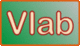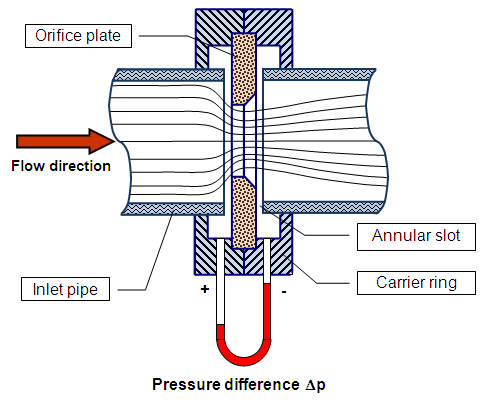online_headloss_orifice_meter:  Head loss using an orifice meterAn orifice meter
 ν = f(T) ReDo = 4Q/(πDoν) K = f(ReDo, Do/D1) Ao = (π/4) Do2 hf = [Q/(KAo)]2/(2g) h = hf/[(ρ2/ρ1) - 1]

[SI units: Q in m3/s; D in m; T in °C; h in m]
[U.S. units: Q in cfs; D in ft; T in °F; h in ft]

INPUT DATA:

Select:

 SI units (metric) U.S. Customary units

Discharge Q :

Pipe diameter D1 :

Orifice diameter Do :

Temperature T :

Ratio ρ21 :

[Leave blank to set mercury manometer
as default ρ21 = 13.6]

OUTPUT:

hρ2 =  0

hρ1 =  0

Your request was processed at  09:52:32 pm on June 30th, 2022   [ 220630  21:52:32 ].

Thank you for running online_discharge_orifice_meter.